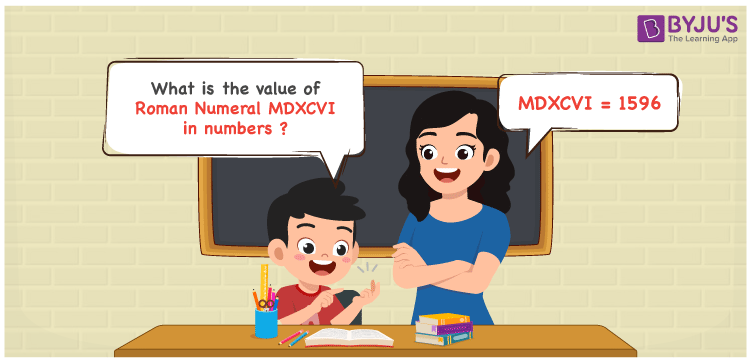MDXCVI Roman Numerals is 1596. This article contains the steps used to transform MDXCVI in numbers as 1596. The roman numerals conversion articles are designed with care by the teachers having a good knowledge of the fundamental concepts in Maths. Therefore, the roman numerals MDXCVI is written as 1596 in numbers.

 Number Roman Numeral 1596 MDXCVI## How to Write MDXCVI Roman Numerals in Numbers?

This section aims to offer students with a proper knowledge of the expansion used to convert the roman numerals into numbers.

MDXCVI = M + D + (C – X) + V + I

MDXCVI = 1000 + 500 + (100 – 10) + 5 + 1

MDXCVI = 1596

## Video Lesson on Roman Numerals## Frequently Asked Questions on MDXCVI Roman Numerals

### Why is MDXCVI the roman numeral of 1596?

We know that

M is the roman numerals of 1000

D is the roman numerals of 500

XC is the roman numerals of 90

VI is the roman numerals of 6

So MDXCVI is the roman numeral of 1596.

### Determine 1600 – 4.

We know that

1600 – 4 = 1596

Hence, 1600 – 4 is 1596 which is written as MDXCVI.

### Find the remainder if MDXCVI is divided by IX.

We know that

MDXCVI = 1596

IX = 9

The remainder if MDXCVI is divided by IX is III.